# ISEE Lower Level Math : Geometry

## Example Questions

### Example Question #2 : How To Find The Area Of A Rectangle

Robert needs to determine the area of his rectangular wall in order to buy the proper amount of paint at the store. When he measures his wall he determines that it is 12 feet high and 20 feet wide. If one can of paint covers 40 square feet of wall, how many cans of paint does Robet need?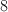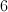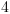Explanation:

Find the area of the wall -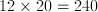Divide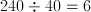6 cans of paint.

### Example Question #1 : How To Find The Area Of A Rectangle

What is the area of a rectangle with a length offeet and a width offeet?square feetsquare feetsquare feet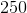square feetsquare feetsquare feet

Explanation:

To find the area of a rectangle, multiply the length by the width.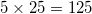### Example Question #11 : How To Find The Area Of A Rectangle

Joe has a rectangular poster that is 3 feet long and 2 feet wide. What is the area of the poster?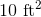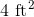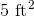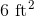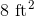Explanation:

The area of a rectangle is found by multiping the length times the width. Given that the length is 3 feet, the width is 2 feet, the total cubic area would be found using this equation: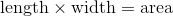Here is the equation with the appropriate numbers plugged in from the question: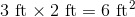6 square feet, oris the correct answer.

### Example Question #12 : How To Find The Area Of A Rectangle

Leslie has a blanket that is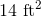in area. Jen has a blanket that is half the size of Leslie's blanket. How big is Jen's blanket?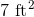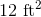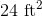Explanation:

If Jen's blanket is half as big as Leslie's blanket, and Leslie's blanket is, then we need to divide by 2 to find the area of Jen's blanket.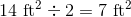The area of Jen's blanket is.

### Example Question #21 : Quadrilaterals

What is the area (in square feet) of a rectangle with a length offeet and a width offeet?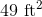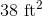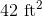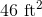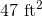Explanation:

The area of a rectangle is calculated by multiplying length times width.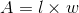Given that the width is 6 feet and the height is 7, the product of 6 and 7 is 42.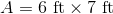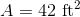Therefore, 42 is the correct answer.

### Example Question #21 : Quadrilaterals

If the area of a rectangle is 28 square inches and its width is 4 inches, what is the length in inches?None of theseExplanation:

The area of a rectangle is equal to the width multiplied by the length, or:If the area of a rectangle is 28 inches and the width is 4 inches, then plugging this into the equation we would get: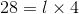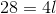Divide both sides by 4 to solve.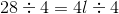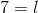### Example Question #22 : Quadrilaterals

If a rectangle has an area of 18, which of the following are possible dimensions of the length and width?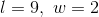None of these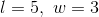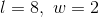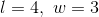Explanation:

If the area of the rectangle is 18, that means that the length and the width, when multiplied together, should equal 18.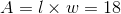The only numbers from the answer choices that would result in the product of 18 are 9 by 2, which is therefore the correct answer.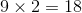### Example Question #16 : How To Find The Area Of A Rectangle

Ben is making a sandbox with a width of 4 feet and a length of 6 feet. What is the area of the sandbox?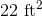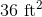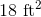Explanation:

The area of a rectangle is the width times the length.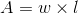The width is 4 feet and the length is 6 feet. Because 4 times 6 is 24, the area is 24 square feet.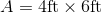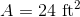### Example Question #11 : How To Find The Area Of A Rectangle

Jerry has a mat with an area of 20 square feet and a length of 5 feet. What is the width of the mat in inches?

None of these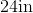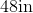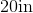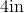Explanation:

The area of a rectangle is the width times the length.Given that the area is 20 square feet and the length is 5 feet, the width would have to be 4 feet because 5 times 4 is 20.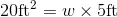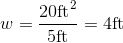Given that the question asks for the width in inches, 4 should be multiplied by 12 (as there are 12 inches in a foot).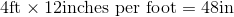This gives us a product of 48 inches, which is the width.

### Example Question #41 : Geometry

If the length of a rectangle is 2r and the width of the rectangle is 3w, what is the area?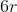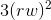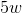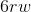The area of a rectangle is found by multiplying the length by the width. Given that the length is 2r and that the length is 3w, the area will be the product of those numbers, which is.